## Spring pendulum

 Flow* spring_pendulum.model

## Model description

We study the behavior of the planar spring-pendulum described in . It consists of a solid ball of mass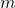and a spring of natural length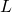. The spring constant is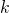.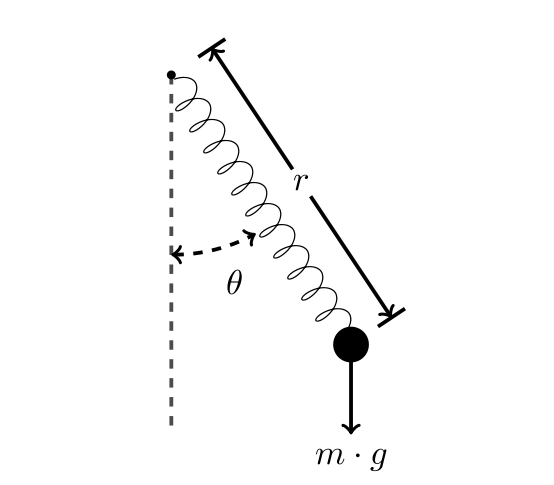We study the evolutions of the length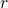of the spring and the angle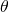between the spring and the vertical. They are modeled by the following differential equations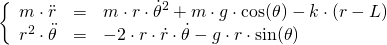which can be equivalently translated to the first-order ODE as below.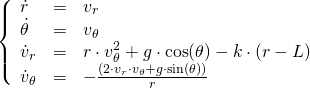The constants are set as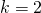,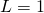, and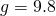.

## Reachability settings

We consider the initial set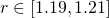,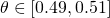,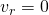and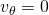.

## Results

The following figure shows an overapproximation computed by Flow* for the time horizon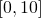.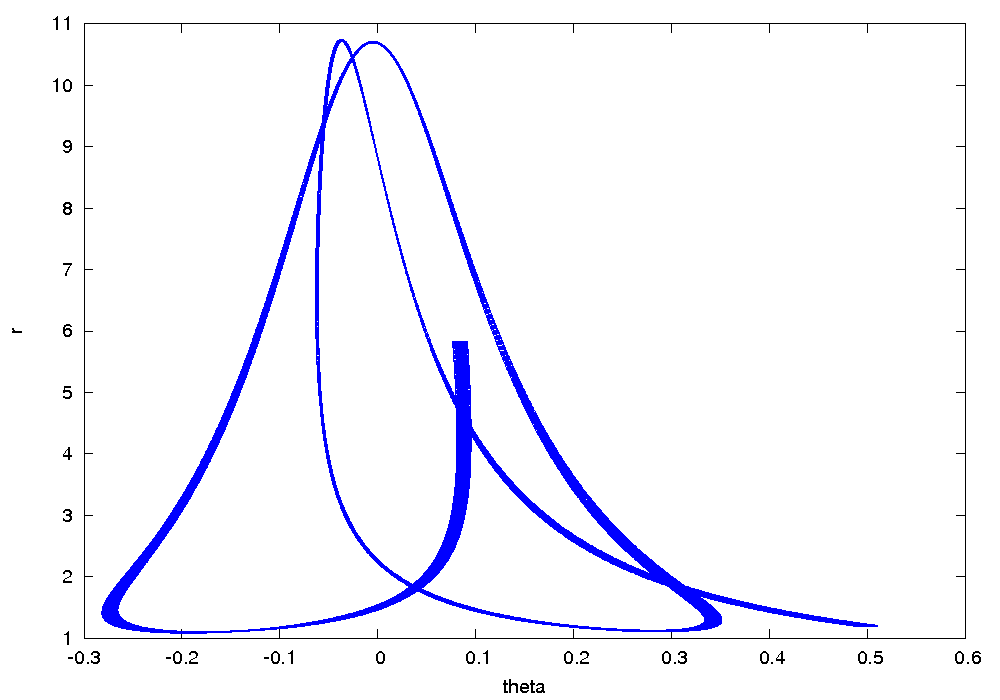## References

 J. D. Meiss. Differential Dynamical Systems (Monographs on Mathematical Modeling and Computation), Book 14, SIAM publishers, 2007.